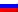UDC 621.921.27:620.186.82

# THE DEPENDENCE OF THE MEAN AND VARIANCE PERIMETER AND AREA OF THE PROJECTION OF THE SILICON CARBIDE GRINDING POWDERS’ GRAIN FRACTIONS ON THE SIZE OF THE PASS MESH

Nosenko Vladimir Andreevich1, Aleksandrov Aleksey Aleksandrovich2
1Volzhsky Polytechnic Institute (branch) of Volgograd State Technical University, Doctor of Technical Sciences, Professor

Abstract
Silicon carbide black grinding powders 54C grits F36-F180, mass-produced at Volzhsky Abrasive Plant, screened into fractions according to GOST R 52381. From each fraction obtained grains sampled by quartering, and then perimeter and area of the grain projection profile on a horizontal plane was measured in a specially designed program for processing electronic images. The results were processed using the methods of mathematical statistics - test hypotheses about the distribution law, variance and regression analysis. It was established that in the majority of samples grain area and perimeter in fractions Q3 - Q5 obeys the log-normal distribution, which is by the number of fractions of about 70%, and the mass fractions of grain - about 90%. The dependence of the mean and variance of the average perimeter and area from the control screens of the cell size inside the grits obeys a linear dependence from passage mesh. Mean perimeter and area of the grain fractions of different grits, with the same number of screens passage, connected with the mesh size of the screen, respectively, by linear-proportional and power dependence. The dependence variance of the perimeter and the area of the grain size fractions from the pass cell screen was approximated by power functions.

Category: 05.00.00 Technical sciences

Article reference:
The dependence of the mean and variance perimeter and area of the projection of the silicon carbide grinding powders’ grain fractions on the size of the pass mesh // Modern scientific researches and innovations. 2017. № 1 [Electronic journal]. URL: http://web.snauka.ru/en/issues/2017/01/76769View this article in Russian